< Back to TunerCodeSA Index
ACCEL/DECEL Tab

[-] ASYNC ACCEL

Description
Asynchronous accel enrichment are extra sequential injector firings that occur due to rapid throttle opening. If the throttle is opened at a rate greater than
ACCEL dTPS DEADBAND and the RPM is between MINIMUM RPM and MAXIMUM RPM, an accel pump event is triggered.

Async Accel IPW (AAIPW) Calculation:
If overrun fuel cut is inactive:
AAIPW = async_accel_base_ipw_ x async_accel_dtps_factor_ x async_accel_load_factor_

If overrun fuel cut is active:
AAIPW = async_accel_base_ipw_ x async_accel_fuel_cut_dtps_factor_

The active injector size factor is then applied to AAIPW.

Once all injectors_fired_count injectors have been fired, the async accel event is over and AAIPW is set to 0. Another async accel event cannot be triggered until the throttle opening rate drops below ACCEL dTPS DEADBAND.

ASYNC PARAMETERS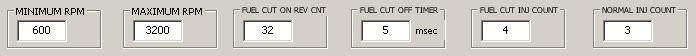• MINIMUM RPM - Minimum RPM for async accel event to be triggered.
• MAXIMUM RPM - Maximum RPM for async accel event to be triggered.

• FUEL CUT ON REV CNT - Fuel cut must be active this number of engine revolutions in order to use the "fuel cut is active" AAIPW calculation.
• FUEL CUT OFF TIMER - When fuel cut deactivates, the "fuel cut is active" AAIPW calculation will remain in use for this amount of time.

• FUEL CUT INJ COUNT - Sets injectors_fired_count when fuel cut is active>.
• NORMAL INJ COUNT - Sets injectors_fired_count when fuel cut is inactive>.

BASE IPW ECT TABLE• BASE IPW ECT TABLE - Sets async_accel_base_ipw_ for AAIPW calculation. AAIPW is higher at lower ECT.

ASYNC FACTOR TABLES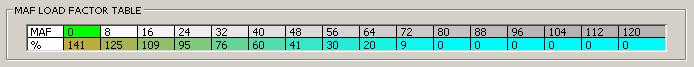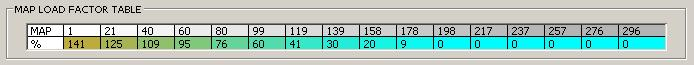NORMAL dTPS FACTOR TABLE• NORMAL dTPS FACTOR TABLE - Sets async_accel_dtps_factor_ for AAIPW calculation. AAIPW is higher at faster throttle opening rates.

FUEL CUT dTPS FACTOR TABLE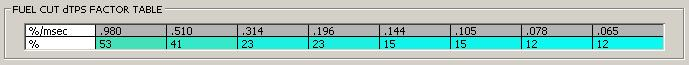• FUEL CUT dTPS FACTOR TABLE - Sets async_accel_fuel_cut_dtps_factor_ for AAIPW calculation. AAIPW is higher at faster throttle opening rates.

[-] SYNC ACCEL

Description
Synchronous accel adds additional enrichment to the normal calculated injector pulse width (IPW) when the throttle is opening and/or the MAP is increasing.

ACCEL PARAMETERS

dTPS ACCEL PARAMETERS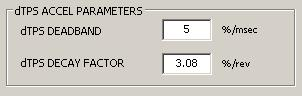dMAP ACCEL PARAMETERSdTPS ACCEL

dTPS ACCEL Description
"Accel dTPS Parameters" control synchronous injector enrichment due to throttle opening rate. If the throttle is opened at a rate greater than
ACCEL dTPS DEADBAND, and accel_dtps_load_factor_ is at least dTPS MIN LOAD FACTOR, an accel dTPS enrichment event is triggered and accel_dtps_TIF_ is calculated. Once the initial value of accel_dtps_TIF_ is calculated, subsequent calculations update accel_dtps_rate_factor and accel_dtps_tps_factor only. In other words, accel_dtps_base_tif_, accel_dtps_rpm_factor_, and accel_dtps_load_factor_ are not updated for subsequent calculations during the dTPS event. The accel_dtps_rate_factor is maximized such that subsequent calculations can only increase the value of accel_dtps_TIF_. This accounts for the case where the rate of throttle opening is increasing.

The accel_dtps_TIF_ calculation:
accel_dtps_TIF_ = accel_dtps_base_tif_ x accel_dtps_rpm_factor_ x accel_dtps_load_factor_ x
accel_dtps_rate_factor x accel_dtps_tps_factor

Once accel_dtps_TIF_ has been set, it is decayed by subtracting accel_dtps_decay every engine revolution.

dTPS ACCEL FACTOR TABLES
Factors from each table are multiplied together with the factor from one of the dTPS ACCEL LOAD FACTOR TABLES to set the total dTPS accel enrichment factor.

dTPS BASE TIF ECT TABLE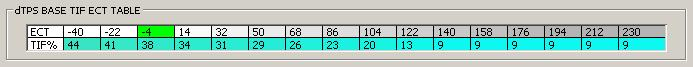• dTPS BASE TIF ECT TABLE - Sets accel_dtps_base_tif_. Enrichment is reduced with increasing ECT.

dTPS TPS FACTOR TABLE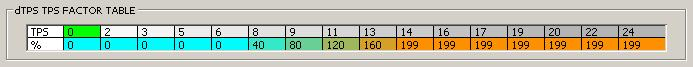• dTPS TPS FACTOR TABLE - Sets accel_dtps_tps_factor. Enrichment is increased at larger throttle openings.

dTPS RATE FACTOR TABLE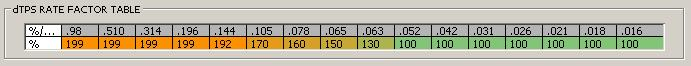• dTPS RATE FACTOR TABLE - Sets accel_dtps_rate_factor. Enrichment is increased with faster throttle opening rates.

dTPS RPM FACTOR TABLE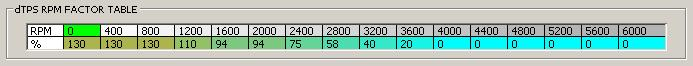• dTPS RPM FACTOR TABLE - Sets accel_dtps_rpm_factor_. Enrichment is decreased at higher RPM.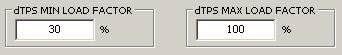• dTPS MIN LOAD FACTOR - The accel_dtps_load_factor_ must be at least this value in order to trigger a dTPS accel event.
• dTPS MAX LOAD FACTOR - Sets the maximum value of accel_dtps_load_factor_ for the accel_dtps_TIF_ calculation. This means that even if the accel_dtps_load_factor_ from one of the tables is greater than this, this value is used in the calculation.

The factor from one of these tables, based on active load source, is included in the multiplication from the
dTPS ACCEL FACTOR TABLES.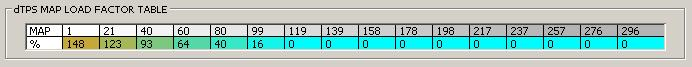• dTPS MAP LOAD FACTOR TABLE - Sets accel_dtps_load_factor_ when MAP (or TPS) is the active load source. Enrichment is increased at lower load.

dMAP ACCEL

dMAP ACCEL Description
If
Enable dMAP Fuel Trim is set, "Accel dMAP Parameters" control enrichment due to the rate of change in intake manifold pressure (dMAP). If MAP is increasing at a rate greater than dMAP DEADBAND, accel_dmap_TIF_ is calculated. Unlike accel_dtps_TIF_, subsequent accel_dmap_TIF_ calculations recalculate all parameters each pass and set accel_dmap_TIF_ only if greater than the current value.

The accel_dmap_TIF_ calculation:
accel_dmap_TIF_ = accel_dmap_base_tif_ x accel_dmap_rpm_factor_ x accel_dmap_load_factor_ x
accel_dmap_rate_factor_

Once accel_dmap_TIF_ has been set, it is decayed by subtracting accel_dmap_decay every engine revolution.
NOTE: accel_dmap_TIF_ is applied ONLY when the active load source is MAP.

dMAP BASE TIF ECT TABLE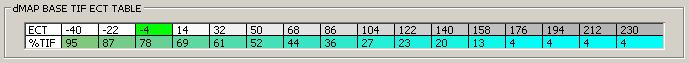• dMAP BASE TIF ECT TABLE - Sets accel_dmap_base_tif_ based on ECT.dMAP RATE FACTOR TABLE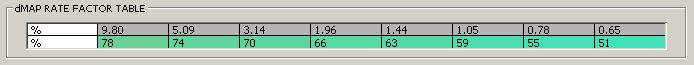dMAP RPM FACTOR TABLE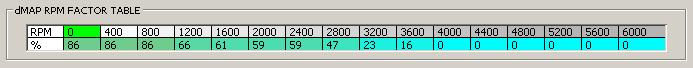• dMAP RPM FACTOR TABLE - Sets accel_dmap_rpm_factor_ based on RPM.

[-] DECEL

Description
Decel reduces the normal calculated injector pulse width (IPW) when the throttle is closing and/or the MAP is decreasing.

DECEL PARAMETERS

dTPS DECEL PARAMETERS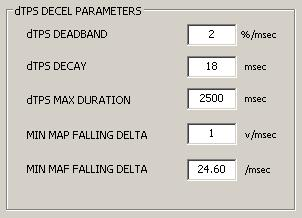• dTPS DEADBAND - The throttle must be closing at a rate greater than this in order to trigger a dTPS decel event.
• dTPS DECAY - Subtracted every 10msec to decay decel_dtps_TIF_.
• dTPS MAX DURATION - This is the maximum amount of time allowed per decel dTPS activation.
• MIN MAP FALLING DELTA - Sets min_load_falling_delta when MAP (or TPS) is active load source.
• MAX MAF FALLING DELTA - Sets min_load_falling_delta when MAF is active load source.

dMAP DECEL PARAMETERS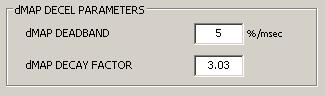dTPS DECEL

DECEL dTPS Description
"Decel dTPS Parameters" control IPW reduction due to throttle closing rate. If the throttle is closing at a rate greater than DECEL dTPS DEADBAND, and engine load is falling at a rate greater than min_load_falling_delta per 10msec, the current throttle position is checked. If TPS is below decel_dtps_mid_tps, decel dTPS is activated. Also, if TPS is between decel_dtps_mid_tps and decel_dtps_max_tps, and the TPS closing rate is above decel_dtps_mid_tps_deadband, decel dTPS is activated.

The decel_dtps_TIF_ calculation:
decel_dtps_TIF_ = decel_dtps_base_tif_ x decel_dtps_rpm_factor_ x

If the newly calculated value is above the current value, decel_dtps_TIF_ is set to the new value. decel_dtps_TIF_ is decayed by subtracting DECEL dTPS DECAY every 10msec. When the DECEL dTPS MAX DURATION timer has expired, or decel_dtps_TIF_ has decayed to 0, decel dTPS is deactivated and decel_dtps_TIF_ is cleared.

dTPS DECEL FACTOR TABLES
Factors from each table are multiplied together with the factor from one of the dTPS DECEL TPS FACTOR TABLES to set the total dTPS decel factor.

dTPS ECT FACTOR TABLE• dTPS ECT FACTOR TABLE - Sets decel_dtps_base_tif_ based on ECT.• dTPS MAP LOAD FACTOR TABLE - Sets decel_dtps_load_factor_ when MAP (or TPS) is active load source.dTPS RPM FACTOR TABLE• dTPS RPM FACTOR TABLE - Sets decel_dtps_rpm_factor_.

dTPS TPS FACTOR

dTPS DECEL TPS FACTOR TABLES
The factor from one of these tables is included in the multiplication from the dTPS DECEL FACTOR TABLES.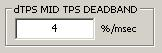dTPS MAX TPS RPM TABLE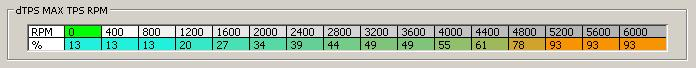• dTPS MAX TPS RPM TABLE - Sets decel_dtps_max_tps based on RPM.

dTPS MID TPS RPM TABLE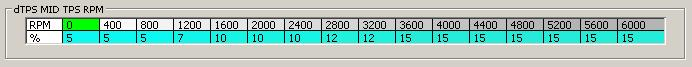• dTPS MID TPS RPM TABLE - Sets decel_dtps_mid_tps based on RPM.

dMAP DECEL

dMAP DECEL Description
If
Enable dMAP Fuel Trim is set, "Decel dMAP Parameters" control IPW reduction due to the rate of change in intake manifold pressure (dMAP). If MAP is decreasing at a rate greater than DECEL dMAP DEADBAND, decel_dmap_TIF_ is calculated. Subsequent decel_dmap_TIF_ calculations recalculate all parameters each pass and set decel_dmap_TIF_ only if greater than the current value.

The decel_dmap_TIF_ calculation:
decel_dmap_TIF_ = decel_dmap_base_tif_ x decel_dmap_rpm_factor_ x

Once decel_dmap_TIF_ has been set, it is decayed by subtracting decel_dmap_decay every engine revolution. (See DECEL dMAP DECAY FACTOR)
NOTE: decel_dmap_TIF_ is applied ONLY when the active load source is MAP.

dMAP DECEL FACTOR TABLES
Factors from each table are multiplied together to set decel_dmap_TIF_.

dMAP BASE TIF ECT TABLE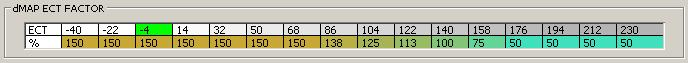• dMAP BASE TIF ECT TABLE - Sets decel_dmap_base_tif_ based on ECT.dMAP RPM FACTOR TABLE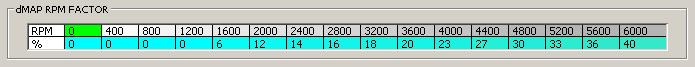• dMAP RPM FACTOR TABLE - Sets decel_dmap_rpm_factor_ based on RPM.

dMAP RATE FACTOR TABLE[-] OVERRUN FUEL CUT

Description
When the throttle closes, if the RPM is above fuel_cut_on_rpm for fuel_cut_on_delay_timer msec, the Fuel Cut flag is set and fuel cut transition (2 injectors cut) is activated for fuel_cut_transition_timer msec. When fuel_cut_transition_timer expires, full fuel cut is activated (4 injectors cut). When the RPM falls to fuel_cut_transition_rpm, fuel cut transition is re-activated (2 injectors cut). When the RPM falls to fuel_cut_off_rpm, fuel cut is deactivated and the Fuel Cut flag is cleared.

If ENABLE A/C CONTROL is set and the AC Switch is ON, the fuel_cut_off_rpm is set to FUEL CUT OFF A/C ON RPM.

If the RPM is falling faster than FUEL CUT RPM Falling DELTA LIMIT, RPM COMP is added to both fuel_cut_off_rpm and to fuel_cut_transition_rpm. This turns fuel cut off at a higher RPM.

When Fuel Cut flag is set, ignition timing is retarded by FUEL CUT RETARD degrees, which is then decayed at 1 degree/cycle during fuel cut transition.

FUEL CUT RPM TABLES

FUEL CUT ON RPM TABLE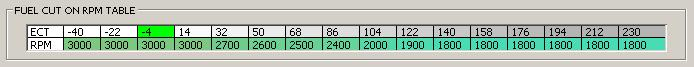• FUEL CUT ON RPM TABLE - Sets fuel_cut_on_rpm based on ECT.

FUEL CUT TRANSITION RPM TABLE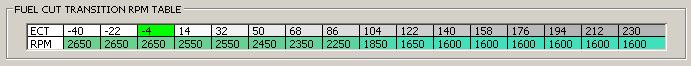• FUEL CUT TRANSITION RPM TABLE - Sets fuel_cut_transition_rpm based on ECT.

FUEL CUT OFF RPM TABLE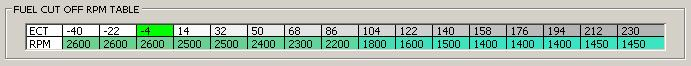• FUEL CUT OFF RPM TABLE - Sets fuel_cut_off_rpm based on ECT.

FUEL CUT PARAMETERS• FUEL CUT TIMER SELECT ECT - When ECT is below this ECT FUEL CUT BELOW ECT TIMERS are used.
• FUEL CUT OFF A/C ON RPM - When the AC Switch is on, this is the minimum fuel_cut_off_rpm.
• FUEL CUT RETARD - When fuel cut is active, the ignition timing is retarded by this number of degrees. When fuel cut deactivates, the retard is decayed at 1 degree/cycle.

FUEL CUT BELOW ECT TIMERS• ON DELAY TIMER - Sets fuel_cut_on_delay_timer when ECT is below FUEL CUT TIMER SELECT ECT.
• TRANSITION TIMER - Sets fuel_cut_transition_timer when ECT is below FUEL CUT TIMER SELECT ECT.

FUEL CUT ABOVE ECT and ABOVE RPM TIMERS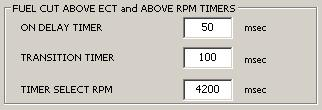• ON DELAY TIMER - Sets fuel_cut_on_delay_timer when ECT is above FUEL CUT TIMER SELECT ECT and RPM is above TIMER SELECT RPM.
• TRANSITION TIMER - Sets fuel_cut_transition_timer when ECT is above FUEL CUT TIMER SELECT ECT and RPM is above TIMER SELECT RPM.
• TIMER SELECT RPM - Sets the RPM for selecting the "above" and "below" RPM fuel_cut_on_delay_timer and fuel_cut_transition_timer timers.

FUEL CUT ABOVE ECT and BELOW RPM TIMERS• ON DELAY TIMER - Sets fuel_cut_on_delay_timer when ECT is above FUEL CUT TIMER SELECT ECT and RPM is below TIMER SELECT RPM.
• TRANSITION TIMER - Sets fuel_cut_transition_timer when ECT is above FUEL CUT TIMER SELECT ECT and RPM is below TIMER SELECT RPM.

FUEL CUT RPM Falling Parameters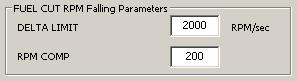• DELTA LIMIT - Sets the RPM falling rate for RPM COMP fuel cut OFF RPM increase.
• RPM COMP - If RPM is falling faster than DELTA LIMIT rpm/sec, this value is added to both fuel_cut_off_rpm and to fuel_cut_transition_rpm.

< Back to TunerCodeSA Index